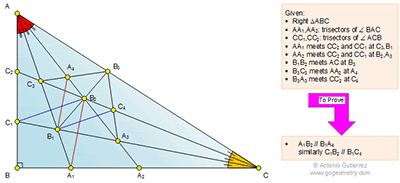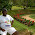## Saturday, May 2, 2015

### Geometry Problem 1117: Right Triangle, Angle Trisection, 90 Degrees, Parallel Lines

Geometry Problem. Post your solution in the comments box below.
Level: Mathematics Education, High School, Honors Geometry, College.

Click the diagram below to enlarge it.#### 2 comments:

1.http://s27.postimg.org/m6mecvwgz/pro_1117.png
Per the result of problem 368 we have
B3C3 is an angle bisector of ∠ (AB3B1) => A4 is incenter of triangle AB1B3
And B1A4 is an angle bisector of ∠( AB1B3)
Per the result of problems 1114 and 1116 we have 60 degrees angles and 30 degrees angles as shown on the attached sketch ( see sketch )
So ∠ (A4B1B2)= ∠ (B1B2A1) = 30 degrees => B1A4//A1B2
Similarly we will have C1B2// B1C4

2.Kindly refer my proofs for Problems 1114 - 6.

A4 is the in centre of Tr. AB1B3 so B1A4 bisects the 60 deg angle AB1B3. So A4B1 // A1B2

Similarly B2C1 // B1C4 considering that C4 is the in centre of Tr. B1B3C

Sumith Peiris
Moratuwa
Sri Lanka